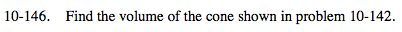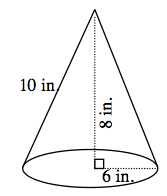### Home > MC2 > Chapter 10 > Lesson 10.2.6 > Problem10-146

10-146.From problem 10-143, you learned that the volume of a coneis one-third the volume of a cylinder.

The equation for the volume of a cylinder is:
Volume = (area of base) (height)
So the volume of a cone is:

$\text{Volume } =\; \frac{1}{3}\left(\text{area of base}\right)\left(\text{height}\right)$

The base is a circle with a radius of 6 inches. So using the equation for area of a circle:
Area of base = (6²)π= 36π

The height of the cylinder is 8 inches. Substitute the known values into the volume equation for a cone:

$\text{Volume}=\frac{1}{3}\left(36\pi \right)\left(8\right)$

Now simplify to get the answer.